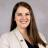# Beef Carcass Yield Grades: What do they mean and how are they calculated?

Updated January 21, 2022#### Christina Bakker

Assistant Professor and SDSU Extension Meat Science Specialist

Beef carcass yield grades (YG) are used to estimate cutability, which is the amount of boneless, closely trimmed retail cuts from the round, loin, rib and chuck a carcass will yield. The system uses a numeric scale of 1 to 5; YG 1 carcasses have the highest, most-desirable cutability and YG 5 carcasses have the lowest, least-desirable cutability. There are four carcass characteristics that factor into a YG equation: hot carcass weight (HCW), ribeye area (REA), backfat thickness (BF) and kidney, pelvic and heart fat (KPH).

• Hot carcass weight is the weight of a carcass as it is leaving the harvest area, before entering a cooler to be chilled. This number can usually be found on the identification tag of the carcass.
• Ribeye area is reported in square inches and is measured on the ribeye muscle that is exposed when a carcass is ribbed. (Ribbing a carcass is the process of cutting through the backbone of the side of beef between the 12th and 13th rib. This cut separates the forequarter from the hindquarter of the carcass.) The REA can be measured using a ribeye grid, which is made of a transparent plastic and has 0.1 square-inch boxes with dots in the middle. To measure the REA, place the grid on the ribeye and count how many dots are touching the ribeye muscle. Make sure you don’t count the dots touching the muscles surrounding the ribeye, and that you are counting dots, not squares. Every ten dots equal one square-inch.
• Backfat thickness is measured in inches using a small ruler. The proper place to measure backfat thickness is ¾ of the length up the ribeye from the backbone end, perpendicular to the outside surface of the carcass.
• Kidney, pelvic and heart fat is an estimation of the percentage of HCW made up by the fat in the kidney, pelvic and heart areas. This is the most-subjective measurement factored into a yield grade, but most carcasses in the Midwest have between 2-3.5% KPH.

Yield grades can be calculated based on the following equation:

• Yield Grade = 2.5 + (2.5 × BF) + (0.2 × % KPH) + (0.0038 × HCW) - (0.32 × REA)

Calculate the values within the parenthesis first and then add or subtract as necessary.

If you do not have a calculator on hand, YGs can be calculated using a series of adjustments based on BF, REA, KPH and HCW.

### Step 1

Backfat is converted into a preliminary YG (PYG) value. Preliminary YG measurements start at 2.0, and 0.25 is added to the PYG measurement for every 0.1 inches of backfat (Table 1).

#### Table 1

Backfat (inches) PYG
0 2.0
.2 2.5
.4 3.0
.6 3.5
.8 4.0
1.0 4.5
1.2 5.0
1.4 5.5

### Step 2

Determine the required REA based on HCW. A 600-pound HCW requires a 11.0 in.2 REA. For every 25 pound increase in HCW, the required ribeye area increases by 0.3 in.2. For each 1 in.2 more than the required REA, subtract 0.3 from the PYG. For every 1 in.2 less than the required REA, add 0.3 to the PYG. Below are two tables to help determine required REAs.

#### Table 2.1

HCW (lbs.) Required REA (in.2)
600 11.0
650 11.6
700 12.2
750 12.8
800 13.4
850 14.0
900 14.6
950 15.2
1000 15.8

#### Table 2.2

HCW (lbs.) Required REA (in.2)
600-608 11.0
609-616 11.1
617-224 11.2
225-633 11.3

### Step 3

Adjust for % KPH. KPH measurements start at 3.5%, and for every 0.5% above 3.5%, add 0.1, and for every 0.5% below 3.5%, subtract 0.1 (Table 3).

#### Table 3

4.5 +0.2
4.0 +0.1
3.5 0
3.0 -0.1
2.5 -0.2
2.0 -0.3
1.5 -0.4
1.0 -0.5

Final YG values for industry purposes are always rounded down, so a calculated YG of 3.9 is still a YG 3 carcass.

Below is an example of how to calculate a YG using this method.

## Example

Carcass Characteristics:
Backfat = 0.4 inches, HCW = 700 lbs., Actual REA = 14.0 in.2, KPH = 2%

• PYG = 3.0
• Required REA = 12.2
• Ribeye adjustment = 12.2 - 14.0 = -1.8 × 0.3 = -0.54